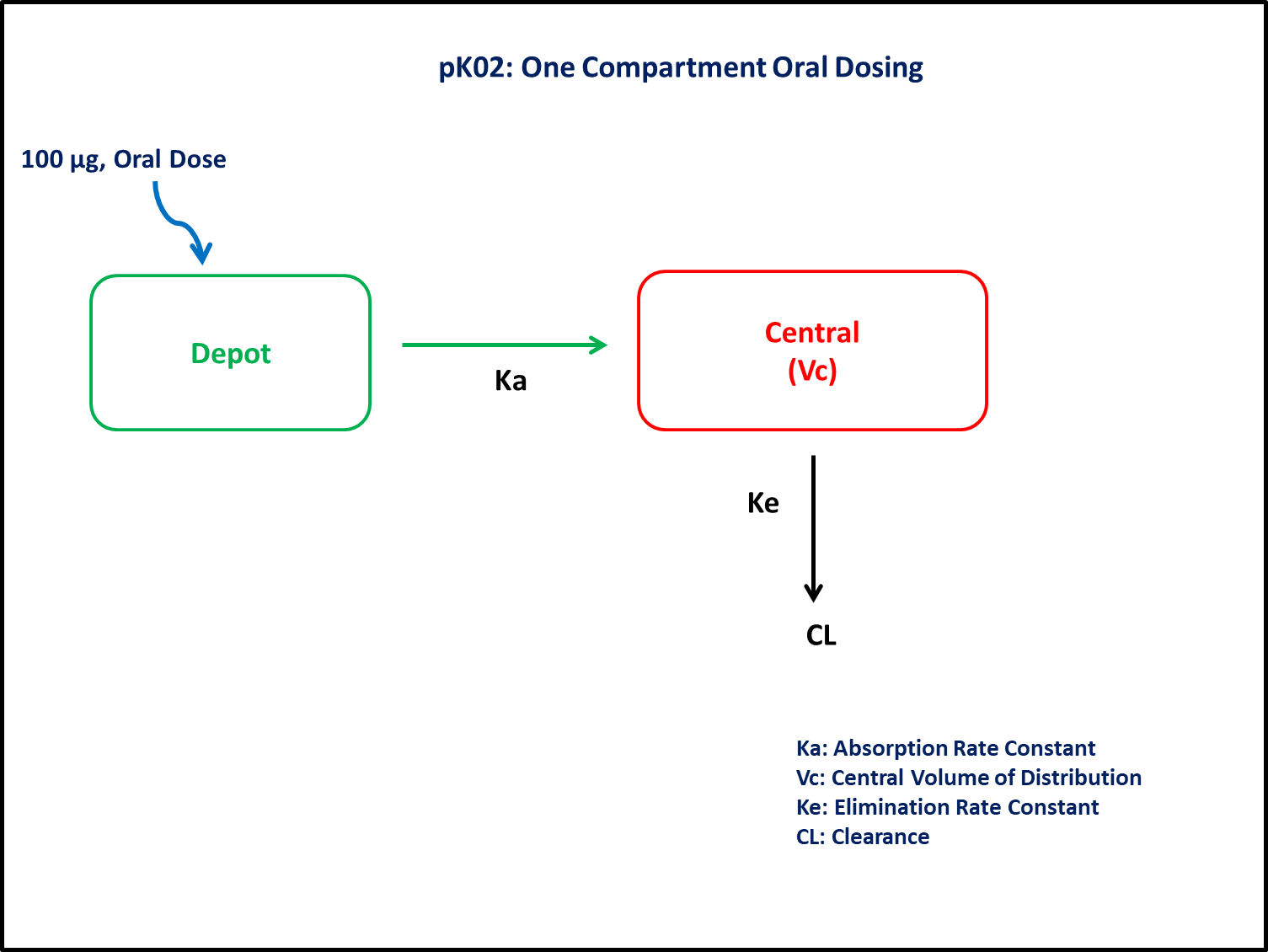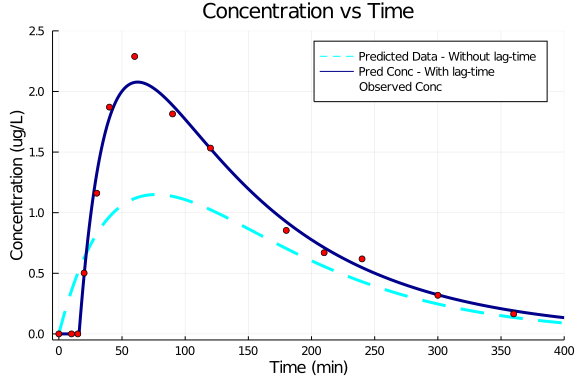# Exercise PK2 - One Compartment Oral Dosing

### Background

• Structural model - 1 compartment with first order absorption (without/with Lag time)

• Route of administration - Oral

• Dosage Regimen - 100 μg Oral

• Number of Subjects - 1### Learning Outcome

By the application of the present model, we will learn how to simulate model for first order input model with and without lag-time.

### Objectives

In this exercise you will learn how to

• Simulate an Oral One Compartment (without/ with lag-time). Assuming oral bioavailability of 100%. The interpretation V includes bioavailability (i.e., it is really estimating V/F).

• Write a differential equation for a one-compartment model

### Libraries

call the "necessary" libraries to get start.

using Pumas
using Plots
using CSV
using StatsPlots
using Random


### Model

In this one compartment model, we administer dose in Depot compartment at time= 0.

pk_02        = @model begin
@param begin
tvka     ∈ RealDomain(lower=0)
tvkel    ∈ RealDomain(lower=0)
tvvc     ∈ RealDomain(lower=0)
tvlag    ∈ RealDomain(lower=0)
Ω        ∈ PDiagDomain(4)
σ²_prop  ∈ RealDomain(lower=0)
end

@random begin
η        ~ MvNormal(Ω)
end

@pre begin
Ka       = tvka * exp(η)
Kel      = tvkel * exp(η)
Vc       = tvvc * exp(η)
lags     = (Depot=tvlag * exp(η),)
end

@dynamics begin
Depot'   = -Ka*Depot
Central' =  Ka*Depot - Kel*Central
end

@derived begin
cp       = @. Central/Vc
dv       ~ @. Normal(cp, sqrt(cp^2*σ²_prop))
end
end

PumasModel
Parameters: tvka, tvkel, tvvc, tvlag, Ω, σ²_prop
Random effects: η
Covariates:
Dynamical variables: Depot, Central
Derived: cp, dv
Observed: cp, dv


### Parameters

The compound follows a one compartment model, in which the various parameters are as mentioned below:

• Ka - Absorption Rate Constant (min⁻¹)

• Kel - Elimination Rate Constant(min⁻¹)

• Vc - Central Volume of distribution (L)

• tlag - Lag-time (min)

• Ω - Between Subject Variability

• σ - Residual error

param1 = (tvka    = 0.013,
tvkel   = 0.013,
tvvc    = 32,
tvlag   = 0,
Ω       = Diagonal([0.0,0.0,0.0,0.0]),
σ²_prop = 0.015)

(tvka = 0.013, tvkel = 0.013, tvvc = 32, tvlag = 0, Ω = [0.0 0.0 0.0 0.0; 0
.0 0.0 0.0 0.0; 0.0 0.0 0.0 0.0; 0.0 0.0 0.0 0.0], σ²_prop = 0.015)

param2 = (tvka    = 0.043,
tvkel   = 0.0088,
tvvc    = 32,
tvlag   = 16,
Ω       = Diagonal([0.0,0.0,0.0,0.0]),
σ²_prop = 0.015)

(tvka = 0.043, tvkel = 0.0088, tvvc = 32, tvlag = 16, Ω = [0.0 0.0 0.0 0.0;
0.0 0.0 0.0 0.0; 0.0 0.0 0.0 0.0; 0.0 0.0 0.0 0.0], σ²_prop = 0.015)


### Dosage Regimen

In this section the Dosage regimen is mentioned:

• Oral dosing of 100 μg at time=0 for a single subject

ev1  = DosageRegimen(100,time=0,cmt=1)
sub1 = Subject(id=1,events=ev1)

Subject
ID: 1
Events: 1


### Simulation

Let's simulate for plasma concentration after oral administration, without lag-time.

#### Without lag-time

Random.seed!(123)
sim_sub1 = simobs(pk_02,sub1,param1,obstimes=0:1:400)
df1      = DataFrame(sim_sub1);


#### With lag-time

Let's simulate for plasma concentration after oral administration, with lag-time.

Random.seed!(123)
sim_sub2 = simobs(pk_02,sub1,param2,obstimes=0:1:400)
df2      = DataFrame(sim_sub2);


### Dataframe & Plot

df_lag = filter(x -> x.time in [0,10,15,20,30,40,60,90,120,180,210,240,300,360], df2)
@df df1 plot(:time, :cp,
label="Predicted Data - Without lag-time",  xlabel="Time (min)", ylabel="Concentration (ug/L)",
title="Concentration vs Time",
color=[:aqua], linestyle=[:dash], linewidth=3,
xticks=[0,50,100,150,200,250,300,350,400], yticks=[0.0,0.5,1.0,1.5,2.0,2.5], xlims=(-5,400), ylims=(-0.05,2.5))
@df df2 plot!(:time, :cp,
label="Pred Conc - With lag-time",
color=[:darkblue], linewidth=3)
@df df_lag scatter!(:time,:dv,
label="Observed Conc", color=[:red])